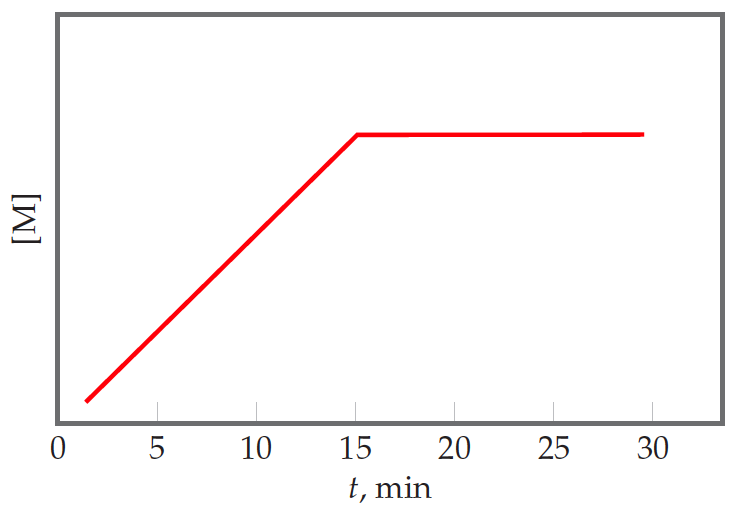# Problem: Suppose that for the reaction K + L → M, you monitor the production of M over time, and then plot the followinggraph from your data:Is the reaction occurring at a constant rate from t = 0 to t = 15 min?

⚠️Our tutors found the solution shown to be helpful for the problem you're searching for. We don't have the exact solution yet.

###### Problem Details

Suppose that for the reaction K + L → M, you monitor the production of M over time, and then plot the following